Home > PC > Chapter 11 > Lesson 11.1.1 > Problem11-14

11-14.
1. Mike has two toy boats. The first travels at a constant rate of 10 ft/sec. The second accelerates so that it has a rate of t ft/sec. Homework Help ✎

1. Sketch speed vs. time graphs for each boat.

2. How far has each boat traveled after 5 seconds?

3. How far will they have traveled after t seconds?

4. When will the second boat pass the first?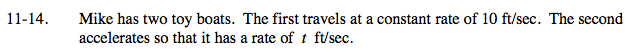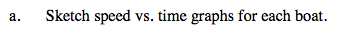Put time on the x-axis and speed (or rate) on the y-axis.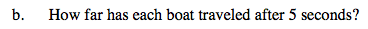Distance = (rate)(time), or using your graph
it is the area under the curve.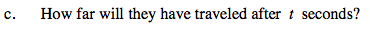First boat: d = 5(t)
Second boat: d = 0.5(t)(t)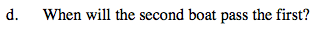When 5t = 0.5t².